Courses

# Static Random Access Memory (SRAM) Electrical Engineering (EE) Notes | EduRev

## VLSI System Design

Created by: Machine Experts

## Electrical Engineering (EE) : Static Random Access Memory (SRAM) Electrical Engineering (EE) Notes | EduRev

The document Static Random Access Memory (SRAM) Electrical Engineering (EE) Notes | EduRev is a part of the Electrical Engineering (EE) Course VLSI System Design.
All you need of Electrical Engineering (EE) at this link: Electrical Engineering (EE)

Objectives

In this lecture you will learn the following

• SRAM Basics
• CMOS SRAM Cell
• CMOS SRAM Cell Design
• WRITE Operation

28.1 SRAM Basics

The memory circuit is said to be static if the stored data can be retained indefinitely, as long as the power supply is on, without any need for periodic refresh operation. The data storage cell, i.e., the one-bit memory cell in the static RAM arrays, invariably consists of a simple latch circuit with two stable operating points. Depending on the preserved state of the two inverter latch circuit, the data being held in the memory cell will be interpreted either as logic '0' or as logic '1'. To access the data contained in the memory cell via a bit line, we need atleast one switch, which is controlled by the corresponding word line as shown in Figure 28.11.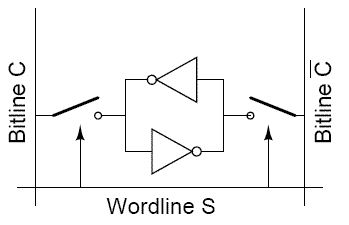Fig 28.11: SRAM Cell

28.2 CMOS SRAM Cell

A low power SRAM cell may be designed by using cross-coupled CMOS inverters. The most important advantage of this circuit topology is that the static power dissipation is very small; essentially, it is limited by small leakage current. Other advantages of this design are high noise immunity due to larger noise margins, and the ability to operate at lower power supply voltage. The major disadvantage of this topology is larger cell size. The circuit structure of the full CMOS static RAM cell is shown in Figure 28.12. The memory cell consists of simple CMOS inverters connected back to back, and two access transistors. The access transistors are turned on whenever a word line is activated for read or write operation, connecting the cell to the complementary bit line columns.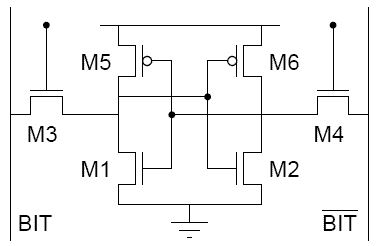Fig 28.21: Full CMOS SRAM cell

28.3 CMOS SRAM Cell Design

To detemine W/L ratios of the transistors, a number of design criteria must be taken into consideration. The two basic requirements, which dictate W/L ratios, are that the data read operation should not destroy the stored information in the cell. The cell should allow stored information modification during write operation. In order to consider operations of SRAM, we have to take into account, the relatively large parasitic column capacitance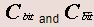and column pull-up transistors as shown in Figure 28.31.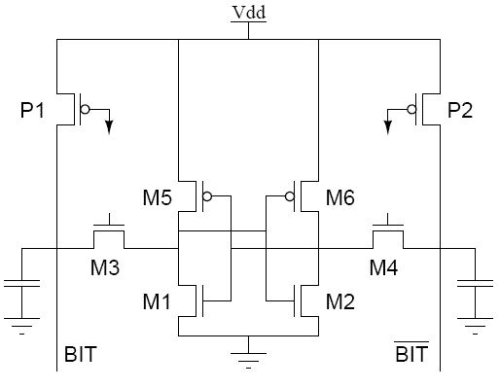Fig 28.31: CMOS SRAM cell with precharge transistors

When none of the word lines is selected, the pass transistors M3 and M4 are turned off and the data is retained in all memory cells. The column capacitances are charged by the pull-up transistors P1 and P2. The voltages across the column capacitors reach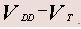Consider a data read operation, shown in Figure 28.41, assuming that logic '0' is stored in the cell. The transistors M2 and M5 are turned off, while the transistors M1 and M6 operate in linear mode. Thus internal node voltages are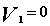and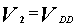before the cell access transistors are turned on. The active transistors at the beginning of data read operation are shown in Figure 28.41.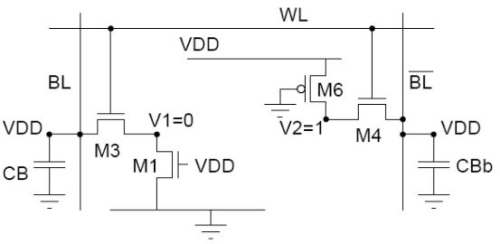After the pass transistors M3 and M4 are turned on by the row selection circuitry, the voltage of CBb will not change any significant variation since no current flows through M4. On the other hand M1 and M3 will conduct a nonzero current and the voltage level of Cwill begin to drop slightly. The node voltage V1 will increase from its initial value of '0'V. The node voltage V1 may exceedthe threshold voltage of M2 during this process, forcing an unintended change of the stored state.

Therefore voltage V1 must not exceed the threshold voltage of M2, so the transistor M2 remains turned off during read phase, i.e.,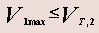(Eq 28.1)

The transistor M3 is in saturation whereas M1 is linear, equating the current equations we get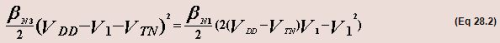substituting Eq 28.1 in Eq 28.2 we get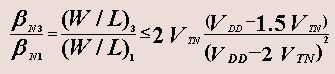(Eq 28.3)

28.5 WRITE Operation

Consider the write '0' operation assuming that logic '1' is stored in the SRAM cell initially. Figure 28.51 shows the voltage levels in the CMOS SRAM cell at the beginning of the data write operation. The transistors M1 and M6 are turned off, while M2 and M5 are operating in the linear mode. Thus the internal node voltage V1  = VDD and  v2 = 0before the access transistors are turned on. The column voltage Vb is forced to '0' by the write circuitry. Once M3 and M4 are turned on, we expect the nodel voltage vto remain below the threshold voltage of M1, since M2 and M4 are designed according to Eq. 28.1.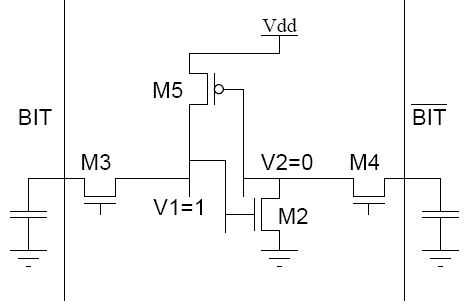Fig 28.51: SRAM start of write '0'

The voltage at node 2 would not be sufficient to turn on M1. To change the stored information, i.e., to force V1 = 0 and V1  = VDD, the node voltage V1 must be reduced below the threshold voltage of M2, so that M2 turns off. When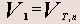the transistor M3 operates in linear region while M5 operates in saturation region. Equating their current equations we get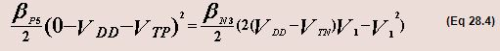Rearranging the condition of Vin the result we get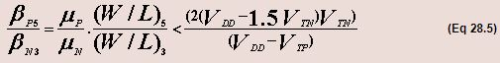28.6 WRITE Circuit

The principle of write circuit is to assert voltage of one of the columns to a low level. This can be achieved by connecting either BIT or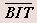to ground through transistor M3 and either of M2 or M1. The transistor M3 is driven by the column decoder selecting the specified column. The transistor M1 is on only in the presence of the write enable signal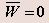and when the data bit to be written is '0'. The transistor M2 is on only in the presence of the write signaland when the data bit to be written is '1'. The circuit for write operation is shown in Figure 28.61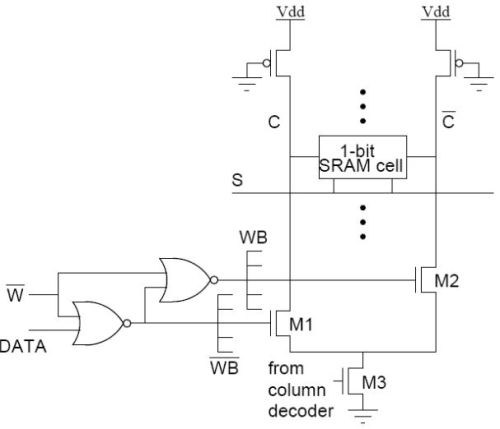Fig 28.61: Circuit for write operation

Offer running on EduRev: Apply code STAYHOME200 to get INR 200 off on our premium plan EduRev Infinity!

,

,

,

,

,

,

,

,

,

,

,

,

,

,

,

,

,

,

,

,

,

;